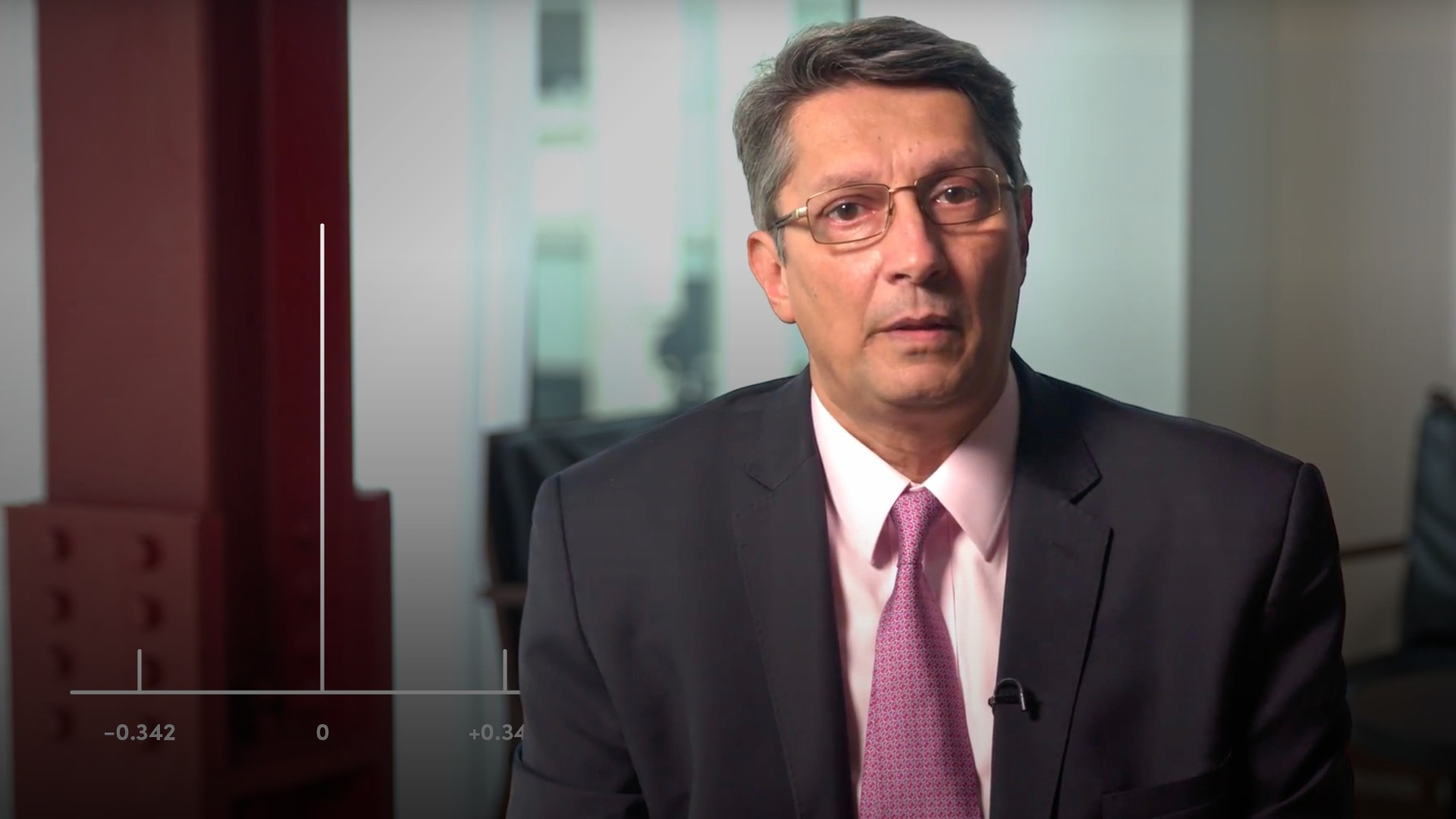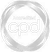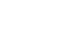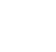# Lognormal Probability Distributions### Abdulla Javeri

In this video of the series on probability distributions, Abdulla explains the basic characteristics of lognormal distributions and why it is used in preference to the normal distribution.

Financial MathsIn this video of the series on probability distributions, Abdulla explains the basic characteristics of lognormal distributions and why it is used in preference to the normal distribution.

Speak to an expert

Speak to an expert today to access this and all of the content on our platform.### Lognormal Probability Distributions

6 mins 44 secs

Overview

Lognormal distributions are often used in financial markets due to their allowance of negative returns and non-negative asset prices. A brief explanation and review of logs can be found below.

Key learning objectives:

• Understand why logs are used in financial markets

• Define the differences between a lognormal distribution and a normal distribution

## Speak to an expert

Speak to an expert today to access this and all of the content on our platform.

Summary

#### What is a lognormal distribution?

A log is the power, or exponent that a base number needs to be raised by to get to another number. For example, log to the base 10 of 100 is 2, because the base number 10, raised to the power of 2 (10 squared) equals 100. In a natural log, the base number ‘e’ Eulers’ constant is 2.71 something. So, log to the base e of 100 is the power that e needs to be raised by to get to a 100, and it happens to be around 4.61.

#### How can this be shown mathematically?

Log(e) of 100 = 4.60517, e^4.60617 = 100

#### Why are logs used more often than normal distributions in financial markets?

In a lognormal distribution, the numbers on the x axis are natural logs and they are normally distributed. In other words there’s an equal probability of the log being 0.342 as it is of it being -0.342.

A lognormal distribution allows for large negative returns and non-negative assets prices. That’s why it tends to be used more often in financial markets than the normal distribution, although we often assume normal distributions, which is fine if the time frame is relatively short.

#### How do we calculate the continuously compounded rate?

If we have a number which we call PV, and we continuously compound it, we get to another number which is FV. If we know what both PV and FV are, we can solve for the continuously compounded rate. It’s the natural log of FV over PV divided by t, time. For our purposes the continuously compounded rate is the same as the natural log. So the log is the continuously compounded rate of return. Applying that to a lognormal distribution what we can say is the probability of a positively compounded rate is the same as the probability of a negatively compounded rate of the same amount which we can then convert into prices.

#### What is the Formula to calculate ‘FV’ and ‘r’?

• PV * exp^(rxt)  = FV
• r = Ln(FV/PV)/t

## Speak to an expert

Speak to an expert today to access this and all of the content on our platform.### Abdulla Javeri

Abdulla’s career in the financial markets started in 1990 when he entered the trading floor of the London International Financial Futures Exchange, LIFFE, and qualified as a pit trader in equity and equity index options. In 1996, Abdulla became a trainer for regulatory qualifications and then for non-exam courses, primarily covering all major financial products.

There are no available videos from "Abdulla Javeri"

### Accredited by